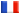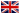# Séminaire de Cryptographie

## Robert Carls### Explicit Serre-Tate theory and applications

This talk is about joint work with David Lubicz. By a classical result of Serre and Tate the deformation space of an ordinary abelian variety is given by a formal torus. In Serre-Tate coordinates the problem of canonical lifting is trivial. Unfortunately, in general it is difficult to compute the Serre-Tate parameters of a given abelian variety. Alternatively, one may use canonical coordinates which are induced by a canonical theta structure. Mumford introduced theta structures in order to construct an arithmetic moduli space of abelian varieties. We apply a multi-variate Hensel lifting procedure to a certain set of p-adic theta identities which are obtained using Mumford's formalism of algebraic theta functions. As an application we give a point counting algorithm for ordinary abelian varieties over a finite field which is quasi-quadratic in the degree of the finite field.